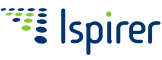Please, note, that Ispirer SQLWays 6.0 is no longer supported and provided to clients.
Check out the relevant toolkit documentation.

# Ispirer SQLWays Database Migration Software

#### DECIMAL, NUMERIC, NUMERICSA and NUMERICSTS

Numeric data types with fixed precision and scale.

DECIMAL(p,s)

p (precision) specifies the maximum total number of decimal digits that can be stored, both to the left and to the right of the decimal point. The precision must be a value from 1 through 64.

s (scale) specifies the maximum number of decimal digits that can be stored to the right of the decimal point. Scale must be a value from 0 through p.

Precision and scale must be specified.

The DECIMAL data type is stored internally as a packed decimal number with two decimal digits per byte.

NUMERIC(p,s)

The NUMERIC data type is similar to DECIMAL. The maximum precision is 15.

NUMERIC values are stored as ASCII strings, right justified with leading zeros. Each digit occupies one byte internally. The embedded sign has an EBCDIC value.

NUMERICSA(p,s)

The NUMERICSA data type (sometimes called NUMERIC SIGNED ASCII) is a COBOL data type that is the same as the NUMERIC data type, except that the embedded sign has an ASCII value instead of an EBCDIC value.

The maximum precision is 15.

NUMERICSTS(p,s)

The NUMERICSTS data type (sometimes called SIGN TRAILING SEPARATE) is a COBOL data type that is the same as the NUMERIC data type, except that the sign is saved in a separate byte.

The maximum precision is 14.